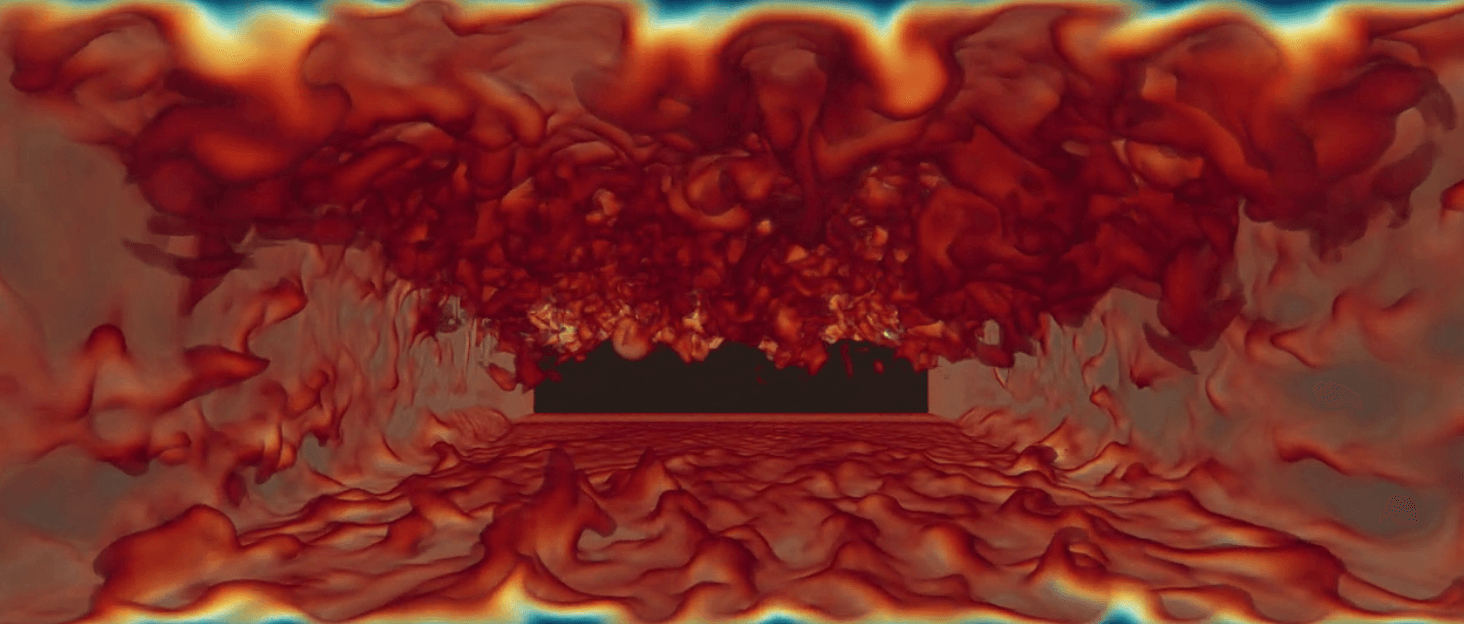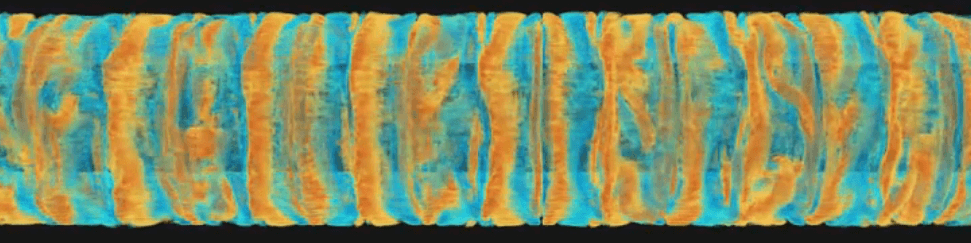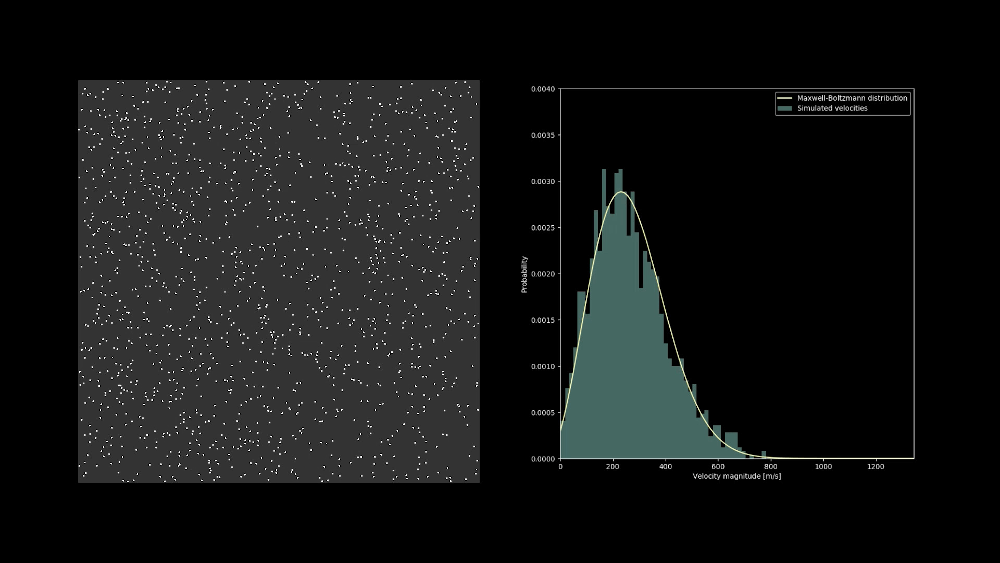### » Just-in-time volumetric CFD visualization

July 24, 2020 at 14:15 | Adrian Kummerländer

For my seminar talk I wrote another LBM solver as a literate Org-document using CUDA and SymPy. The main focus was on just-in-time volumetric visualizations of the simulations performed by this code. While this is not ready to publish yet, check out the following impressions:Further videos are available on my YouTube channel. e.g. a Taylor-Couette flow:### » Visualizing the velocity distribution of a hard sphere gas

March 24, 2020 at 21:42 | Adrian Kummerländer

The velocity distribution of a system of colliding hard sphere particles quickly evolves into the Maxwell-Boltzmann distribution. One example of this surprisingly quick process can be seen in the following video:### » Visualize the sky for arbitrary time and space coordinates on earth

January 11, 2020 at 21:12 | firmament | 071bc3 | Adrian Kummerländer

The primary color of the sky is caused by Rayleigh and Mie scattering of light in the atmosphere. While attending the lecture on Mathematical Modelling and Simulation at KIT I implemented a ray marcher to visualize this. Together with a model for calculating the sun direction for given coordinates and times this allows for generating interesting plots:For more details check out Firmament.

### » Started working on a framework for generating LBM kernels

October 27, 2019 at 22:35 | Adrian Kummerländer

During the past exam season I now and then continued to play around with my GPU LBM code symlbm_playground. While I mostly focused on generating various real-time visualizations using e.g. volumetric ray marching, the underlying principle of generating OpenCL kernels from a symbolic description has not lost its promise.

This is why I have now started to extract the generator part of this past project into a more general framework. Currently boltzgen targets C++ and OpenCL using a shared symbolic description while providing various configuration options:

λ ~/p/d/boltzgen (boltzgen-env) ● ./boltzgen.py --help
usage: boltzgen.py [-h] --lattice LATTICE --layout LAYOUT --precision
PRECISION --geometry GEOMETRY --tau TAU [--disable-cse]
[--functions FUNCTIONS [FUNCTIONS ...]]
[--extras EXTRAS [EXTRAS ...]]
language

Generate LBM kernels in various languages using a symbolic description.

positional arguments:
language              Target language (currently either "cl" or "cpp")

optional arguments:
-h, --help            show this help message and exit
--lattice LATTICE     Lattice type (D2Q9, D3Q7, D3Q19, D3Q27)
--layout LAYOUT       Memory layout ("AOS" or "SOA")
--precision PRECISION
Floating precision ("single" or "double")
--geometry GEOMETRY   Size of the block geometry ("x:y(:z)")
--tau TAU             BGK relaxation time
--disable-cse         Disable common subexpression elimination
--functions FUNCTIONS [FUNCTIONS ...]
Function templates to be generated
--extras EXTRAS [EXTRAS ...]


The goal is to build upon this foundation to provide an easy way to generate efficient code using a high level description of various collision models and boundary conditions. This should allow for easy comparision between various streaming patterns and memory layouts.

### » Symbolically generate D3Q19 OpenCL kernel using SymPy

June 15, 2019 at 20:45 | symlbm_playground | d71fae | Adrian Kummerländer

My recent experiments in using the SymPy CAS library for automatically deriving optimized LBM codes have now evolved to the point where a single generator produces both D2Q9 and D3Q19 OpenCL kernels.

Automatically deriving kernel implementations from the symbolic formulation of e.g. the BGK relaxation operator presents itself as a very powerful concept. This could potentially be developed to the point where a LBM specific code generator could produce highly optimized GPU programs tailored to arbitrary simulation problems.

### » Experimental visualization of the velocity curl

April 28, 2019 at 12:53 | compustream | ecaf66 | Adrian Kummerländer

Calculating the curl of our simulated velocity field requires an additional compute shader step. Handling of buffer and shader switching depending on the display mode is implemented rudimentarily for now. Most of this commit is scaffolding, the actual computation is more or less trivial:

const float dxvy = (getFluidVelocity(x+1,y).y - getFluidVelocity(x-1,y).y)
/ (2*convLength);
const float dyvx = (getFluidVelocity(x,y+1).x - getFluidVelocity(x,y-1).x)
/ (2*convLength);

setFluidExtra(x, y, dxvy - dyvx);


This implements the following discretization of the 2d curl operator:

Let $V : \mathbb{N}^2 \to \mathbb{R}^2$ be the simulated velocity field at discrete lattice points spaced by $\Delta x \in \mathbb{R}_{\gt 0}$. We want to approximate the $z$-component of the curl for visualization:

$\omega := \partial_x V_y - \partial_y V_x$

As we do not possess the actual function $V$ but only its values at a set of discrete points we approximate the two partial derivatives using a second order central difference scheme:

$\overline{\omega}(i,j) := \frac{V_y(i+1,j) - V_y(i-1,j)}{2 \Delta x} - \frac{V_x(i,j+1) - V_x(i,j-1)}{2 \Delta x}$

Note that the scene shader does some further rescaling of the curl to better fit the color palette. One issue that irks me is the emergence of some artefacts near boundaries as well as isolated “single-cell-vortices”. This might be caused by running the simulation too close to divergence but as I am currently mostly interested in building an interactive fluid playground it could be worth it to try running an additional smoothening shader pass to straighten things out.